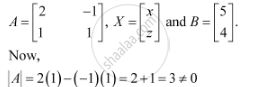Share

# Examine the Consistency of the System of Equations. 2x − Y = 5 X + Y = 4 - CBSE (Science) Class 12 - Mathematics

ConceptApplications of Determinants and Matrices

#### Question

Examine the consistency of the system of equations.

2− y = 5

x + = 4

#### Solution

The given system of equations is:

2− y = 5

x + = 4

The given system of equations can be written in the form of AX = B, where∴ A is non-singular.

Therefore, A−1 exists.

Hence, the given system of equations is consistent.

Is there an error in this question or solution?

#### Video TutorialsVIEW ALL 

Solution Examine the Consistency of the System of Equations. 2x − Y = 5 X + Y = 4 Concept: Applications of Determinants and Matrices.
S